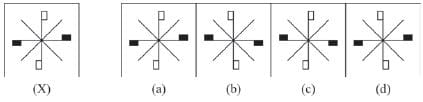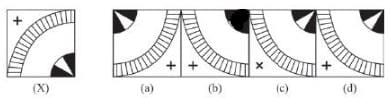# Olympiad Test; Water Images - 1

## 10 Questions MCQ Test Science Olympiad Class 8 | Olympiad Test; Water Images - 1

Description
Attempt Olympiad Test; Water Images - 1 | 10 questions in 20 minutes | Mock test for Class 8 preparation | Free important questions MCQ to study Science Olympiad Class 8 for Class 8 Exam | Download free PDF with solutions
QUESTION: 1

Solution:
QUESTION: 2

Solution:
QUESTION: 3

### Directions : In each of the following questions, you are given a combination of alphabets and/or numbers followed by four alternatives (a), (b), (c) and (d). Choose the alternative which most closely resembles the water-image of the given combination.  RECRUIT

Solution:
QUESTION: 4

Directions : In each of the following questions, you are given a combination of alphabets and/or numbers followed by four alternatives (a), (b), (c) and (d). Choose the alternative which most closely resembles the water-image of the given combination.

ACOUSTIC

Solution:
QUESTION: 5

Directions : In each of the following questions, you are given a combination of alphabets and/or numbers followed by four alternatives (a), (b), (c) and (d). Choose the alternative which most closely resembles the water-image of the given combination.

FAMILY

Solution:
QUESTION: 6

Directions : In each of the following questions, you are given a combination of alphabets and/or numbers followed by four alternatives (a), (b), (c) and (d). Choose the alternative which most closely resembles the water-image of the given combination.

NUCLEAR

Solution:
QUESTION: 7

Directions : In each of the following questions, you are given a combination of alphabets and/or numbers followed by four alternatives (a), (b), (c) and (d). Choose the alternative which most closely resembles the water-image of the given combination.

QUARREL

Solution:
QUESTION: 8

Directions : In each of the following questions, you are given a combination of alphabets and/or numbers followed by four alternatives (a), (b), (c) and (d). Choose the alternative which most closely resembles the water-image of the given combination.

U4P15B7

Solution:
QUESTION: 9

Directions : In the following questions, choose the correct water image of the figure (X) from the given four alternatives.Solution:
QUESTION: 10

Directions : In the following questions, choose the correct water image of the figure (X) from the given four alternatives.Solution:Use Code STAYHOME200 and get INR 200 additional OFF Use Coupon Code